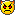Montreal Math ClubMathematics students in Montreal - Étudiants de mathématiques à MontréalHomeCalendarFAQSearchMemberlistUsergroupsRegisterLog in

 Montreal Math Club :: Mathematics :: Problem Solving Share

Writing integers as sums of primesAuthorMessagePosts : 184
Join date : 2009-09-15
Age : 32
Location : the infinite, frictionless plane of uniform densitySubject: Writing integers as sums of primesSun Dec 20, 2009 4:20 pm Include 1 as a prime for this problem (blasphemy!). Show that every integer is the sum of distinct primes.PythagorasPosts : 4
Join date : 2009-09-16Subject: GoldbachThu Jan 14, 2010 11:50 pm This reminds me of another problem. Show that every even integer greater than two can be written as the sum of two primes.Note that they don't have to be distinct and 1 does not count as a prime in this case.Kudos to any of you that solve this problem...Let's say it's a bit of a tough one...Posts : 184
Join date : 2009-09-15
Age : 32
Location : the infinite, frictionless plane of uniform densitySubject: Re: Writing integers as sums of primesFri Jan 15, 2010 2:17 pm Mitch wrote:This reminds me of another problem. Show that every even integer greater than two can be written as the sum of two primes.Note that they don't have to be distinct and 1 does not count as a prime in this case.Kudos to any of you that solve this problem...Let's say it's a bit of a tough one...What's up Mitch! I haven't seen you in a while!The problem you are giving is quite hard indeed! Here's a plot of the number of representations of each even integer as a sum of two primes. It looks very nice!My problem above is easier, rest assured.Subject: Re: Writing integers as sums of primesWriting integers as sums of primesPage 1 of 1
 Similar topics» Writing Christian Fiction - and Getting It Published!
» Went to Payless shoes, couldn't afford \$1.50 to buy them.
» Dear God 6
» Lesson Plan : Back to Nature by Mrs. Kobzili Z.
» she rose and parted the lace curtain ...spiritual writing by Julie Ralphs

Permissions in this forum:You cannot reply to topics in this forumJump to: Select a forum||--Math Club|   |--Mathematical Sundays|   |--Suggestions|   |--Introduce yourself|   |--Mathematics|   |--Problem Solving|   |   |--Solved problems|   |   |   |--Calculus and Analysis|   |--Combinatorics|   |--Geometry|   |--Number Theory|   |--Probabilities and statistics|   |--Set theory and Topology|   |--Online Resources|   |--Chit chat    |--The Water Cooler    |--Mathematical poems